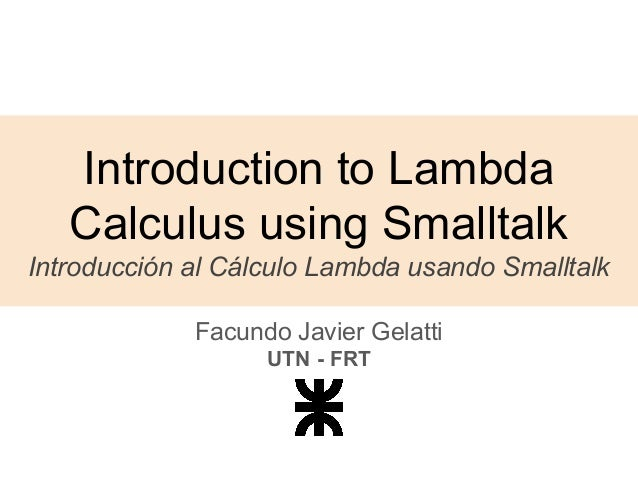Successfully reported this slideshow.Upcoming SlideShare
×

# Introduction to Lambda Calculus using Smalltalk by Facundo Javier Gelatti

173 views

Published on

It can be said that Lambda Calculus is the smallest universal programming language of the world. It consists of a single transformation rule (variable substitution) and a single function definition scheme. It was introduced by Alonzo Church in the 1930s as a way to formalizing the concept of effective computability. Since then, it has influenced many programming languages that we use today, including Smalltalk. Understanding the concepts defined in Lambda Calculus is not only interesting, but also important, because it encodes the basic principles of computing and programming, and it gives you another point of view from which to analyze the nature of computation. In this talk, I will introduce the concept of Lambda Calculus, and show you how some usual values and structures (such as booleans or pairs) can be implemented in Smalltalk using some of those concepts. The Lambda Calculus computation model can be approximated in Smalltalk by only allowing the definition and calling of one-argument blocks. For me, it was enlightening to see how booleans and other structures that I used every day were implemented in Smalltalk only by using objects and messages. I hope I can give that feeling back, by showing you how these concepts can be also implemented only using blocks.

Published in: Technology
• Full Name
Comment goes here.

Are you sure you want to Yes No• Be the first to like this

### Introduction to Lambda Calculus using Smalltalk by Facundo Javier Gelatti

1. 1. Introduction to Lambda Calculus using Smalltalk Introducción al Cálculo Lambda usando Smalltalk Facundo Javier Gelatti UTN - FRT
2. 2. What is Lambda Calculus? ¿Qué es el Cálculo Lambda? How does Lambda Calculus work? ¿Cómo funciona el Cálculo Lambda? Understanding Lambda Calculus using Smalltalk Entendiendo el Cálculo Lambda usando Smalltalk
3. 3. What is Lambda Calculus? ¿Qué es el Cálculo Lambda?
4. 4. Alonzo Church - https://en.wikipedia.org/wiki/File:Alonzo_Church.jpg Alonzo Church 1930s
5. 5. Effective computability Computabilidad efectiva (que algo sea computable) Turing Machines Máquinas de Turing Lambda Calculus Cálculo Lambda Computable Function Función Computable Program (or Algorithm) Programa o algoritmo
6. 6. How does Lambda Calculus work? ¿Cómo funciona el Cálculo Lambda?
7. 7. Lambda Calculus syntax La sintaxis del Cálculo Lambda ● Function definition scheme Esquema de definición de funciones ● Transformation rule Regla de transformación
8. 8. Variable binding operator (function definition) Definición de una función Variable (function parameter) Parámetro de la función Lambda term (function body) Cuerpo de la función λ x.x Lambda Calculus syntax La sintaxis del Cálculo Lambda
9. 9. Function to be applied Función a ser aplicada Function argument Argumento de la función (que puede ser otra función!) f x Lambda Calculus syntax La sintaxis del Cálculo Lambda
10. 10. (λx.x) y β-Reduction
11. 11. (λx.x) y β-Reduction
12. 12. (λx.x) y y β-Reduction
13. 13. (λa.(λb.a)) x y β-Reduction
14. 14. (λa.(λb.a)) x y β-Reduction
15. 15. (λa.(λb.a)) x y (λb.x) y β-Reduction
16. 16. (λa.(λb.a)) x y (λb.x) y β-Reduction
17. 17. (λa.(λb.a)) x y (λb.x) y x β-Reduction
18. 18. How it is possible that we can express anything that is computable using only Lambda Calculus? ¿Cómo es posible que podamos expresar cualquier cosa que sea computable utilizando solamente Cálculo Lambda?
19. 19. Understanding Lambda Calculus using Smalltalk Entendiendo el Cálculo Lambda usando Smalltalk
20. 20. (λx.x) y [:x | x] value: y An approximation Una aproximación
21. 21. We can start to see how can we express computable functions using Lambda Calculus Empezamos a ver cómo podemos expresar funciones computables utilizando el Cálculo Lambda
22. 22. Lambda Calculus is a general model of computation. Understanding the concepts defined in Lambda Calculus is important, because it encodes the basic principles of computing and programming, and it gives you another point of view from which to analyze the nature of computation. Conclusions
23. 23. What we implemented Lo que implementamos ● Booleans (T, F) ● assert* ● not ● and ● or ● pair (first, second)
24. 24. Things that we didn’t cover Cosas que no hicimos ● Lists ● Numbers ● operations with the numbers (plus, minus, times,...) ● loops ● recursion ● ...
25. 25. Resources Recursos para ver más cosas así ● Programming with nothing - Tom Stuart https://codon.com/programming-with-nothing ● Fun with the Lambda Calculus - Corey Haines https://www.youtube.com/watch?v=QPqoFCHpLF4
26. 26. CONTACT Facundo Javier Gelatti javiergelatti@gmail.com Thank you! ¡Gracias! FAST - UNLP